# Statistical analysis of data

Last updated: July 19, 2023

## SummaryStatistical analysis is one of the principal tools employed in epidemiology, which is primarily concerned with the study of health and disease in populations and its clinical applications. Statistics is the science of collecting, analyzing, and interpreting data, and a good epidemiological study depends on statistical methods being employed correctly. At the same time, flaws in study design can affect statistics and lead to incorrect conclusions. Descriptive statistics measure, describe, and summarize features of a collection of data/sample without making inferences that go beyond the scope of that collection/sample. Common measures of descriptive statistics are those of central tendency and dispersion. Measures of central tendency describe the central distribution of data and include the mode, median, and mean. Measures of dispersion describe how data is distributed and include range, quartiles, variance, and deviation. The counterpart of descriptive statistics, inferential statistics, relies on data to make inferences that do go beyond the scope of the data collected and the sample from which it was obtained. Inferential statistics involves parameters such as sensitivity, specificity, positive/negative predictive values, confidence intervals, and hypothesis testing.

The values used to describe features of a sample or data set are called variables. Variables can be independent, in the sense that they are not dependent on other variables and can thus be manipulated by the researcher for the purpose of a study (e.g., administration of a certain drug), or dependent, in the sense that their value depends on another variable and, thus, cannot be manipulated by the researcher (e.g., a condition caused by a certain drug). Variables can furthermore be categorized qualitatively in categorical terms (e.g., eye color, sex, race) and quantitatively in numerical terms (e.g., age, weight, temperature).

Statistical analysis is used in all types of epidemiological studies, including the evaluation of diagnostic tests before approval for clinical practice. These rely on inferential statistics to draw conclusions from sample groups that can be applied to the general population.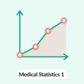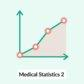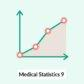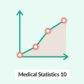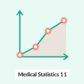## Descriptive and inferential statistics

• Descriptive statistics
• The collection, measurement, summary, and description of data concerning a population or population sample
• Employs measures of central tendency (mode, median, and mean) and of dispersion (range, quartiles, variance, and deviation)
• Inferential statistics: the analysis of data collected from a population sample to make inferences about the population as a whole

## Sample statistics and population parameters

• Sample statistics: descriptive or inferential statistics using data derived from a population sample rather than the population as a whole
• Population parameters: characteristics of a population as a whole

## Measures of central tendency

• Definition: measures to describe a common, typical value of a data set (e.g., clustering of data at a specific value)
• Approach: The type of measure used depends on the sample size.
Measures of central tendency
Measure Definition Example
Mean (statistics)
• The arithmetic average of the data set
• Limitations: affected by extreme values (outliers)
• The sum of all the data divided by the number of values in the data set. (e.g., consider a data set of 3, 6, 11, 14, 16, 19. The mean value is 11.5 (= 69/6).
Median (statistics)
• The middle value of the data set that has been arranged in order of magnitude; it divides the upper half of the data set from the lower half
• Not strongly affected by outliers or skewed data
• Uneven number of values: 3, 6, 11, 16, 19. The median value is the middle value = 11.
• Even number of values: 2, 3, 5, 7, 9, 10. The median value is the average of the two middle values = (5+7)/2 = 6.
Mode (statistics)
• In a data set with the values “3, 6, 6, 11, 11, 11, 2, 2,” the mode = 11.

## Outlier

• Definition: a data point/observation that is distant from other data points/observations in a data set
• Problem
• It is important to identify outliers, because outliers can indicate errors in measurement or statistical anomalies.
• The mean is easily influenced by outliers
• Approach
• Using a trimmed mean: calculate the mean by discarding extreme values in a data set and using the remaining values
• Use the median or mode: useful for asymmetrical data; these measures are not affected by extreme values because they are based on ranks of data (median) or the most commonly occurring value (mode) rather than the average score of all values
• Removing outliers can also distort the interpretation of data. It should be done with caution and with a view to reflecting the respective data set.
• Regression to mean: a phenomenon in which any measurement taken after the measurement of a random variable lying at the extreme (i.e., above or below the mean) is likely to be closer to the mean

## Overview

• Measures of dispersion are statistical measures that describe the variability or spread of data to determine the degree of its homogeneity or heterogeneity.
• For example, two companies with 10 employees each pay the same mean salary of \$50,000, but company A has a range of \$10,000–\$400,000 while company B has a range of \$40,000–\$60,000. In company A, one employee would be earning \$400,000 and another \$10,000, with the remaining 8 earning a mean of \$11,250. Despite paying the same mean salary, the average employee of company B has a higher salary.
• Some measures of dispersion relate to sample distribution, while others relate to sampling distribution. 
Measures of dispersion
Measure Definition Explanation
Range (statistics)
• The difference between the largest and smallest value in a data set
• Sensitive to extreme data values
• Helps to identify an unusually wide or narrow data range, which may occur with data entry errors (e.g., data that actually belongs to another study population)
• In the data set “3, 4, 9, 27,” the range is 24 (i.e., 27-3).
Interquartile range
• The range from the second to the third quartile.
• Calculated by establishing the difference between the 75th and 25th percentile
• Less influenced by extreme data values (outliers)
• Calculated as the difference between the 75th and 25th percentile
Quartile
• One quarter of a data set
• Each quartile includes 25% of the population data set.
• First quartile (lower quartile): 25% of all values are smaller than this value.
• Third quartile (upper quartile): 75% of all values are smaller than this value.
Percentile
• A value on a scale from 1 to 100 indicating the percentage of a distribution equal to or below that value
• Growth charts feature length-for-age and weight-for-age percentiles to help evaluate a child's development in relation to other children their age.
• If a child's weight is in the 25th percentile for his or her age, this child weighs more than 25% of children of the same age group, but less than 75% children of the same age group. For example:
• 3rd percentile (= 3/100-quantile):3% of all values are smaller than this value.
• 50th percentile (= 50/100-quartile): 50% of all values are smaller than this value (median).
• 97th percentile (= 97/100-quartile): 97% of all values are smaller than this value.
• Upper limit of normal (ULN): a value at the upper extreme of the reference range (95th percentile) of the target population
• Lower limit of normal (LLN): a value at the lower extreme of the reference range (5th percentile) of the target population
Variance (statistics)
• The average of the squared deviations from the mean
• Represented by σ2 (S2)
• σ2 = the sum of squared deviations from the mean divided by total number of observations
• Calculated by subtracting the mean from each population data set value. Each difference is then squared and added together. Finally, the total sum is divided by n-1.
Standard deviation (SD)
• The standard deviation is calculated by first calculating the mean. The mean is subtracted from each population data set value. Each difference is squared and added together. The total sum is divided by the total number of data set values -1. The square root of this value is the standard deviation (σ or s).
• 1 SD = 68% of the data set
• 2 SD = 95% of the data set
• 3 SD = 99.7% of the data set
Standard error of the mean (SEM)
• The deviation of the sample mean from the population mean 
• Influenced by the standard deviation (e.g., a greater SD increases the error) and the sample size (a smaller sample size will increase the error)
• True SEM = σ/√(population size) 
• Estimated SEM = s/√(sample size)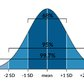## Variables• Definition
• Variables: measured values of population attributes or a value subject to change
• General population: the group from which the units of observation are drawn (e.g., all the patients in a hospital)
• Unit of observation: the individual who is the subject of the study (e.g, inhabitant of a region, a patient)
• Attribute: a character of the unit of observation (e.g., gender, patient satisfaction)
• Attribute value
• Variables can be qualitative (e.g., male/female) or quantitative (e.g., temperature: 10°C, 20°C) in nature
• Quantitative variables can be discrete or nondiscrete (continuous) variables (see “Probability” below).
• Types of variables
• Independent variable: a variable that is not dependent on other variables and can thus be manipulated by the researcher for the purpose of a study
• Dependent variable: a variable with a value that depends on another variable and therefore cannot be manipulated by the researcher
• Types of quantitative variables
• Discrete variable: variables that can only assume whole number values
• Continuous variable (nondiscrete variable): variables that can assume any real number value
• Categorical variable (nominal variable): variables that have a finite number of categories that may not have an intrinsic logical order
• Variable scales
• Definition: types of measurement scales (categorized as categorical scales and metric scales)
• Categorical scale (qualitative)
• Metric scale (quantitative)
Types of scales 
Types Characteristics Measure of central tendency Measure of dispersion Statistical analysis Data illustration
Nominal scale
• Data cannot be ranked
• Mode
• Absolute and relative frequency
• Not applicable
Ordinal scale
• Data can be ranked
Interval scale
• There is no natural zero point.
Ratio scale
• There is a natural zero point.## Normal distribution (Bell curve, Gaussian distribution)

• Normal distributions differ according to their mean and variance, but share the following characteristics:
• The same basic shape
• Unimodal distribution (i.e., one peak)
• Asymptotic to the x-axis
• Symmetry (i.e., a symmetrical bell curve)
• 68% of the data falls within 1 SD of the mean.
• 95% of the data falls within 2 SD of the mean.
• 99.7% of the data falls within 3 SD of the mean.
• Total area under the curve = 1
• All measures of central tendency are equal (mean = median = mode)
• Standard normal distribution (Z distribution): a normal distribution with a mean of 0 and standard deviation of 1## Nonnormal distributions

Types of nonnormal distributions
Description Meaning
Bimodal distribution
• Two subgroups within the study population (e.g., the incidence rate of Hodgkin's lymphoma that has the first peak at 25–30 years and the second peak at 50–70 years)

Positively skewed distribution

(right-skewed distribution)

• The data set has a peak on the left side and a long tail on the right (positive direction).
• The mean falls closer to the right tail.
• Mean > median > mode

Negatively skewed distribution

(left-skewed distribution)

• The data set has a peak on the right side and a long tail on the left (negative direction).
• The mean falls closer to the left tail.
• Mean < median < mode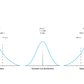## Standard normal value (Z-score, Z-value, standard normalized score)

• Enables the comparison of populations with different means and standard deviations
• Standard normal value = (value - population mean) divided by standard deviation
• A means of expressing data scores (e.g., height in centimeters or meters) in the same metric (specifically, in terms of units of standard deviation for the population)
• Determines how many standard deviations an observation is above or below the mean

## Recommended measures

Recommended measures according to distribution
Distribution Measures of central tendency Measure of spread
Normal (symmetrical)
• Mean
• Median
• Mode
Skewed (asymmetrical)
• Median

## Data illustration

### Categorical data

• Frequency table
• Presents data values for each category in a table
• Illustrates which values in a data set appear frequently
• Pie chart
• Describes the frequency of categories in a circular graph divided into slices, with each slice representing a categorical proportion
• Useful for depicting a small number of categories and large differences between them
• Bar graph
• Describes the frequency of categories in bars separated from each other (the height/length of each bar represents a categorical proportion)
• Useful for depicting many categories of information (compared to a pie chart)
• Frequency can be expressed in absolute or relative terms.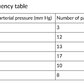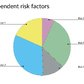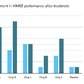### Continuous data

• Histogram
• A histogram is similar to a bar graph but displays data on a metric scale.
• The data is grouped into intervals that are plotted on the x-axis.
• Useful for depicting continuous data
• Similar to a bar chart, but differs in the following ways:
• Used for continuous data
• The bars can be shown touching each other to illustrate continuous data.
• Bars cannot be reordered.
• Box plot
• Quartiles and median are used to display numerical data in the form of a box.
• Useful for depicting continuous data
• Shows the following important characteristics of data:
• Easily shows measures of central tendency, range, symmetry, and outliers at a glance
• Scatter plot
• A graph used to display values for (typically) two variables of data, plotted on the horizontal (x-axis) and vertical (y-axis) axes using cartesian coordinates, which represent individual data values
• Helps to establish correlations between dependent and independent variables
• Helps to determine whether a relationship between data sets is linear or nonlinear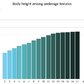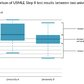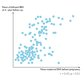## Hypothesis testing

### Types of hypothesis

Two mutually exclusive hypotheses (null hypothesis and alternative hypothesis) are formulated.

• Null hypothesis (H0): The assumption that there is no statistically significant relationship between two measured variables (e.g., the exposure and the outcome) or no significant difference between two studied populations. Statistical tests are used to either reject or accept this hypothesis.
• Alternative hypothesis (H1): : The assumption that there is a relationship between two measured variables (e.g., the exposure and the outcome) or a significant difference between two studied populations. This hypothesis is formulated as a counterpart to the null hypothesis. Statistical tests are used to either reject or accept this hypothesis.
• Directional alternative hypothesis (one-tailed): specifies the direction of a tested relationship
• Non-directional alternative hypothesis (two-tailed): only states that a difference exists in a tested relationship (does not specify the direction)### Interpretation 

• Correct result
• The null hypothesis is rejected when there is a relationship between two measured variables.
• The null hypothesis is accepted when there is no relationship between two measured variables.
• Type 1 error
• Multiple comparisons problem
• When multiple hypotheses are tested simultaneously with one data set (e.g., the data of a trial is tested for different outcomes), the probability of type 1 errors increases:
• P1= 1 - P2
• P1= 1 - (1 - α)m
• P1 = at least one significant result of m test
• P2 = no significant results of m tests; α = type 1 error rate
• Example: when testing 20 hypotheses on one set of data, each at a significance level of α = 0.05, the probability of obtaining at least one significant result by chance can be calculated as follows:
• P(at least one significant result in 20 tests at α = 0.05) = 1 - (1 - 0.05)20)
• P(at least one significant result in 20 tests at α = 0.05) ≈ 1 - 0.36 = 0.64)
• For 20 independent hypotheses that are simultaneously tested on the same data, each at α = 0.05, the probability of a type 1 error in at least 1 test is approximately 64%.
• There are methods to control the type 1 error rate for multiple comparisons; examples include:
• Bonferroni correction: the α value is divided by the number of comparisons performed
• False discovery rate: controls the proportion of false positives among the set of rejected hypotheses
• Results that are not adjusted for multiple comparisons should not be used to infer treatment effects or make clinical decisions to avoid interpreting random results as significant.
• Type 2 error
• Statistical power: (1-β)
• The probability of correctly rejecting the null hypothesis, i.e., the ability to detect a difference between two groups when there truly is a difference
• Complementary to the type 2 error rate
• Positively correlates with the sample size and the magnitude of the association of interest (e.g., increasing the sample size of a study would increase its statistical power)
• Positively correlates with measurement accuracy
• By convention, most studies aim to achieve 80% statistical power.

“The Accusation is POSted But you NEGlect it!” (type I error (Alpha) is a false positive error and type II error (Beta) is false negative error)

Statistical significance” does not mean “clinical significance.”

### Overview of errors

Overview
Statistical test Null hypothesis (H0) is true Null hypothesis (H0) is false
Does not reject H0
• 1-α
Rejects H0
• Power (1-β)## Probability

• Probability of an occurring event (P)
• Describes the degree of certainty that a particular event will take place (e.g., rolling a 6 is considered the event when tossing a die. When throwing a die, the probability of the event 6 occurring is 1/6)
• P = number of favorable outcomes/total number of possible outcomes
• Probability of an event not occurring (Q)
• The degree of certainty that a particular event will not take place (e.g., rolling a 6 is considered the event when tossing a die. When throwing a die, the probability of the event not occurring (rolling a “1”, “2”, “3”, “4”, or “5”) is 5/6)
• Q = number of unfavourable outcomes/total number of possible outcomes OR 1 - P

The actual probability of an event is not the same as the observed frequency of an event.

• Probability of independent events: The probability of event A is not contingent upon the probability of event B and vice versa. (e.g., eye-color and birthdays are two independent variables, with probability distributions independent of each other)
• Conditional probability: the probability of event A occurring given that event B has occurred
• P(A|B) = P(A and B) / P(B)
• P(B) = probability of event B
• P(A and B) = probability of events A and B occurring simultaneously
• Example: the probability of lung cancer in a smoker (A: occurrence of lung cancer; B: occurrence of smoking)
• The underlying condition is that the individual is a smoker: P(B) = probability of being a smoker = number of smokers/total population
• P(A and B) = probability of simultaneously being a smoker and having number of smokers with lung cancer/total population
• Therefore, P(A|B) = the probability of lung cancer arising in a smoker = P(A and B)/P(B) = number of smokers with lung cancer/number of smokers
• Multiplication rule
• P(A and B): the probability of events A and B occurring simultaneously
• The multiplication rule is obtained by rearranging the formula for conditional probability.
• For dependent conditions: P(A and B) = P(B) × P(A|B)
• For independent conditions: P(A and B) = P(B) x P(A)
• The multiplication rule can be applied to a decision tree (a visual representation of all possible outcomes) in order to calculate the probability of one of the branches (a particular outcome).
• Bayes theorem

## Statistical significance vs. clinical significance 

• Significance (epidemiology): the statistical probability that a result did not occur by chance alone
• Statistical significance
• Describes a true statistical outcome (i.e., one that is determined by statistical tests) that has not occurred by chance
• If the statistical significance is high, the probability that the results are due to chance is low.
• Clinical significance (epidemiology)
• Describes an important change in a patient's clinical condition, which may or may not be due to an intervention introduced during a clinical study
• If the clinical significance is high, the intervention is likely to have had a great impact on patient outcome or measures.
• Statistical and clinical significance do not necessarily correlate. A study might have a high statistical significance but the tested intervention did not have any clinical impact on patient outcome.

## Correlation and regression

### Correlation

• Definition
• Example: how does y change if x is changed?
• Interpretation: A correlation coefficient measures the strength (i.e., the degree) and direction (i.e., a positive or negative relationship) of a linear relationship (does not require causality).
• Direction or relationship: can be positive or negative (which are identified by a plus or minus, respectively)
• Strength of relationship

### Regression (epidemiology)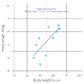## Parametric tests

Parametric tests are used to evaluate statistically significant differences between groups when the study sample has a normal distribution and the sample size is large.

• Pearson correlation coefficient (r)
• Compares interval level variables
• Calculates the estimated strength and direction of a relationship between two variables
• Interpretation
• r is always a value between -1 and 1.
• A positive r-value = a positive correlation
• A negative r-value = negative correlation
• The closer the r-value is to 1, the stronger the correlation between the compared variables.
• The coefficient of determination = r2 (the coefficient may be affected by extreme values and indicates the proportion of a variable's variance that can be predicted by the variance of another variable)
• T-test
• Calculates the difference between the means of two samples or between a sample and population or a value subject to change; especially when samples are small and/or the population or a value subject to change distribution is not known
• Used to determine the confidence intervals of a t-distribution (a collection of distributions in which the standard deviation is unknown and/or the sample size is small)
• One sample t-test
• Interpretation
• The t-value can be classified according a table that lists t-values and their corresponding quantiles based on the number of degrees of freedom (df) and the significance level (α value).
• Alternatively, one may calculate the confidence intervals of the sample observations and check if the population mean (μ0) falls within the range given by the confidence intervals.
• Formula: t-value = (sample mean - population mean)/standard deviation) * √(n)
• Prerequisite: normal distribution (the variance is known and depends on the degrees of freedom.)
• Calculates whether a sample mean differs from the population mean (μ0)
• Two sample t-test
• Calculates whether the means of two groups differ from one another
• Prerequisites
• Both sample groups are drawn from the same population and have the same (but unknown) variance.
• The difference between the observations in the two groups approximately follows a normal distribution.
• Formula: t-value = (mean difference between the two samples/standard deviation) * √(n)
• Interpretation: The t-value is compared with a table of t-values in order to determine whether the difference is statistically significant (similar to the one sample t-test described above).
• Unpaired t-test (independent samples t-test)
• Paired t-test (dependent samples t-test)
• Analysis of variance (ANOVA)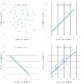T-test has 2 syllables and differentiates between 2 groups; ANOVA has more than 2 syllables and can be used for 2 or more groups.

## Nonparametric tests

Nonparametric tests are used to evaluate the statistically significant difference between groups when the sample has nonnormal distribution and the sample size is small.

• Spearman correlation coefficient
• Calculates the relationship between two variables according to their rank
• Compares ordinal level variables
• Interpretation
• Extreme values have a minimal effect on Spearman coefficient.
• Not precise because not all information from the data set is used.
• See “Correlation.”
• Mann-Whitney U test
• Compares ordinal, interval, or ratio scales
• Calculates whether two independently chosen samples originate from the same population and have identical distributions and/or medians
• Example: comparing two groups of high school students – one with an average GPA of 4.2 and the other an average GPA of 3.2 – to determine if both came from the same larger group.
• Wilcoxon test (rank sum and signed rank)
• Rank sum test: compares the means between groups of different sizes
• Signed rank test: compares the means between pairs of scores that can be matched; substitute for the one-sample t-test when a pre-intervention measure is compared with a post-treatment measure and the null hypothesis is that the treatment has no effect
• Kruskal-Wallis H test
• Extension of the Mann-whitney U test
• Compares multiple groups by testing the null hypothesis (that there is no median difference between at least two groups)
• Binomial test
• Examines whether the observed frequency of an event with binary outcomes (e.g., heads/tails, dead/alive) is statistically probable or not
• Example: if a coin is tossed 20 times, it is likely to land on heads approx. 10 times. If only 9 heads come up, the result is still acceptable.
• If only 6 heads come up (p = 0.04), one is left wondering whether the coin is biased
• From a statistical perspective, the result of a coin toss should be questioned if fewer than 25% of the coin tosses result in heads because the probability of such an event is < 2.5%

## Categorical tests

Categorical tests are used to evaluate the statistically significant difference between groups with categorical variables (no mean values).

• Chi-square test (X2 test)
• Fisher exact test
• Also calculates the difference between the frequencies in a sample but, unlike a Chi-square test, is used when the study sample is small
• Also aims to determine how likely it was the outcomes occurred due to chance

Chi-tegorical:” Chi-square test is used for categorical variables.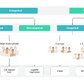## References1. Lee DK, In J, Lee S. Standard deviation and standard error of the mean. Korean J Anesthesiol. 2015; 68 (3): p.220.
2. Harding B, Tremblay C, Cousineau D. Standard errors: A review and evaluation of standard error estimators using Monte Carlo simulations. The Quantitative Methods for Psychology. 2014; 10 (2): p.107-123.
3. What are Categorical, Discrete, and Continuous Variables?. https://support.minitab.com/en-us/minitab-express/1/help-and-how-to/modeling-statistics/regression/supporting-topics/basics/what-are-categorical-discrete-and-continuous-variables/. Updated: January 1, 2018. Accessed: November 17, 2018.
4. Types of Variable. https://statistics.laerd.com/statistical-guides/types-of-variable.php. Updated: January 1, 2018. Accessed: November 17, 2018.
5. Parry T. Statistics in Clinical Research. Inst of Clinical Research ; 2004
6. Interpreting Results of Case-Control Studies. https://www.cdc.gov/training/SIC_CaseStudy/Interpreting_Odds_ptversion.pdf. Updated: October 1, 2014. Accessed: November 20, 2020.
7. Carol K. Redmond, Theodore Colton. Biostatistics in Clinical Trials. Wiley ; 2001: p. 522

##3 free articles remaining

You have 3 free member-only articles left this month. Sign up and get unlimited access.
Evidence-based content, created and peer-reviewed by physicians. Read the disclaimer